## Second Part: Mean and Average Tutorial

In this second part of Mean and Average tutorial , I demonstrate the relationship time-average, delayed-average, moving-average and delayed-moving-average using of average composition diagram and fundamental theorem.

Suppose we have a sequence of measurement that we give notation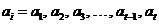. The index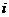of sequence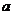is regarded as the position of the element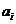. We assume the index of the sequence always start at 1 and increase by natural number up to the last measurement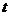. In this case, the sequence have a fixed length. Alternatively, the sequence can also dynamically grow over time. When the sequence grows over time, the lengthis not specified.

The topics are the following:

Resources on Mean and Average